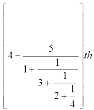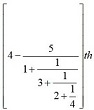# Sample Aptitude Questions of Motorola

1. When n is divided by 6, the remainder is 4. When 2n is divided by 6, the remainder is
1. 2
2. 0
3. 4
4. 1

When n is divided by 6, the remainder is 4. Hence,the number can be written as n = 6k +4
hence, 2n = 12k + 8 and when 2n is divided by 6 : remainder will be ( 12 k + 8 ) / 6 = 2
So,12k gives remainder 0 with 6 and 8 gives remainder 2 with 6. So, remainder is 0 + 2 = 2.Hence, Ans is option A.
2. The value of is 1/20 + 1/30 + 1/42 + 1/56 + 1/72 + 1/90 is
1. 1/10
2. 3/5
3. 3/20
4. 7/20

1/(4×5) + 1/(5×6) + 1/(6×7) + 1/(7×8) + 1/(8×9) + 1/(9×10)
= (1/4 - 1/5) + (1/5 - 1/6) + (1/6 - 1/7) + (1/7 - 1/8) + (1/8 - 1/9) + (1/9 - 1/10)
= 1/4 -1/10 = 3/20
1. Let a = 1/(2 - √3) + 1/(3 - √8) + 1/(4 - √15). Then we have
1. a > 18
2. a >= 18
3. a = 18
4. a = 9

Rationalize the given terms we get
=2+√3 / 22 - (√3)2  + 3 +√8 /32-(√8)2 + 4 + √15 / 42 - (√15)2
=(2+√3)+ (3 +√8)+(4 + √15)
= 9 + (√3+√8+√15)
= 9+8.422 = 17.422
2. The smallest among the numbers 2250, 3150, 5100 and 4200 is
1. 4200
2. 5100
3. 3150
4. 2250

2250, 3150, 5100 and 4200 can be written as :
2250 = ( 2 )5*50 = 3250
3150 = ( 3 )3*50 = 2750
5100 = ( 5 )2*50 = 2550
4200 = ( 4 )4*50 = 25650
Hence, the smallest number is 5100
So, Ans is option B.
3. The greatest of the following numbers 0.16, √0.16, (0.16)2, 0.04 is
1. 0.16
2. √0.16
3. 0.04
4. (0.16)2

Check Each Option
A => 0.16
B => 0.4
C => 0.04
D => (0.16)2 = 0.0256
Hence, the greatest number is 0.4
So, Ans is option B.
4. Ifpart of a journey takes 10 minutes, then to complete ( 3 / 5 )th of that journey, it will take
1. 40 minutes
2. 45 minutes
3. 48 minutes
4. 36 minutespart of journey means = 4 - 155/40 = 5/40th of the journey will take 10 mins
Hence, for the full journey it will take 80 mins
Therfore for 3/5 th of the journey,it will take = ( 3/5 ) * 80 = 48 mins
Hence, Ans is option C.
5. If a number is increased by 25% and the resulting number is decreased by 25%,then the percentage increase or decrease finally is
1. No change
2. Decreased by 61/4%
3. Increased by 61/4%
4. Increased by 6%

Let the number be 100.
So 100 + 25% = 125
125 - 25% = 93.75
So net change = 100 - 93.75 = 6.25%
6. A reduction of 20% in the price of rice enables a customer to purchase 12.5 kg more for Rs. 800. The original price of rice per kg is
1. Rs. 14
2. Rs. 16/-
3. Rs. 12/-
4. Rs. 15/-

Let the price of rice is Rs x / kg
As per the question
800/ 0.8x - 800 / x = 12.5
160/0.8x = 12.5
on solving x= 16
7. In two alloys A and B, the ratio of zinc to tin is 5 : 2 and 3 : 4 respectively. Seven kg of the alloy A and 21 kg of the alloy B are mixed together to form a new alloy. What will be the ratio of zinc and tin in the new alloy?
1. 2:1
2. 1:2
3. 2:3
4. 1:1

Alloy A : which weighted 7 kg has zinc & tin in ratio = 5 : 2 i.e Zinc in alloy A = 5 kg & tin in alloy A = 2kg
Alloy B : which weighted 21 kg has zinc & tin in ratio = 3 : 4 i.e Zinc in alloy A = 9 kg & tin in alloy A = 12kg
Hence, total Zinc : total tin = 14 : 14 = 1 : 1 So, answer is option D.
8. If A : B = 3 : 4 and B : C = 6 : 5, then C : A is
1. 10;9
2. 9:10
3. 8:9
4. 9:8